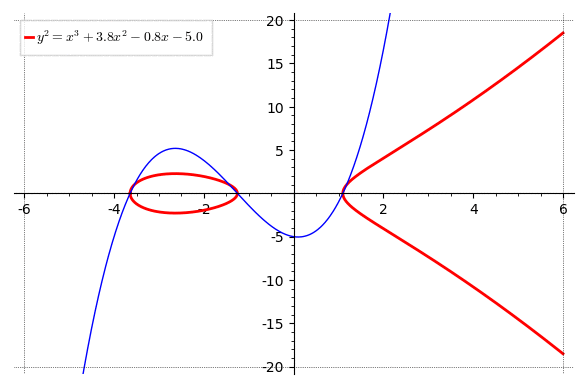# Putting label in the right spot (upper-left) during animationI'm quite new to SageMath. It took some time (half a sunday) to create a code the draws an elliptic curve with a cubic, controlling the speed of the animation and putting a label in. However, I don't know where I can put something like a legend_loc='upper left somewhere or whether that's even possible. My code is as follows:

step=0.2;
var('x,y');
a=animate([
plot(EllipticCurve(y^2==x^3+3.8*x^2-0.8*x+float(k)),xmin=-6,xmax=0
,color="red",thickness=2,legend_label=r'$y^2=x^3+3.8x^2-0.8x+{}$'.format(float(k)))
+plot(EllipticCurve(y^2==x^3+3.8*x^2-0.8*x+float(k)),xmin=10^(-9),xmax=6
,color="red",thickness=2)
+ plot(x^3+3.8*x^2-0.8*x+float(k),xmin=-6,xmax=6,color="blue",thickness=1)
for k in srange(-5,5,step)],xmin=-6,xmax=6,ymin=-20,ymax=20,gridlines=[[-6,6],[-20,20]]);
a.show(delay=1)


I also tried set_legend_options(shadow=False, loc=2) in the plot function, but that doesn't satisfy SageMath either. Anyone an idea how I can put that label at some fixed spot? Btw, it would be even better if I could put that label on the top centre above the animation. I appreciate any suggestions and help.

edit retag close merge delete

Sort by » oldest newest most votedYou have to call set_legend_options on the individual plots after they're created. For simplicity, define a helper function which creates the individual plots (so you can call set_legend_options there):

step=0.2;
var('x,y');
def my_plot(k):
P = plot(EllipticCurve(y^2==x^3+3.8*x^2-0.8*x+float(k)),xmin=-6,xmax=0,color="red",thickness=2,legend_label=r'$y^2=x^3+3.8x^2-0.8x' + ('+' if k >=0 else '') + '{0:.1f}$'.format(float(k)))
P += plot(EllipticCurve(y^2==x^3+3.8*x^2-0.8*x+float(k)),xmin=10^(-9),xmax=6,color="red",thickness=2)
P += plot(x^3+3.8*x^2-0.8*x+float(k),xmin=-6,xmax=6,color="blue",thickness=1)
return P
a=animate([my_plot(k) for k in srange(-5,5,step)],xmin=-6,xmax=6,ymin=-20,ymax=20,gridlines=[[-6,6],[-20,20]]);
a.show(delay=1)I also fixed the legend_label so the number is rounded and it shows + or - correctly.

Btw, it would be even better if I could put that label on the top centre above the animation.

To do this, use the title keyword instead of legend_label.

more

That's true, sorry :( Hopefully you will get a better answer.

This is even better indeed! I must say that I didn't even notice the plus- and minus sign changing at first. Nice solution!

That is quite impressive for half a Sunday! I'd suggest to forget about the legend command and use text. Add the two lines

• text(r'$y^2=x^3+3.8x^2-0.8x{0:+}$'.format(float(k)),(3,-18),fontsize='large', fontweight='bold', color='red')
• text(r'$y=x^3+3.8x^2-0.8x{0:+}$'.format(float(k)),(-3,18),fontsize='large', fontweight='bold', color='blue')

right after the plot of the cubic, ie as separate Graphics objects. I've tried this, the coordinates (3,-18) and (-3,18) put it in a nice spot within your animation. And with the text color, you don't need a little colored line telling the viewer what the label refers to. BUT did you notice the 0:+ instead of your curly braces formatting { } ?? The 0:+ needs curly braces around it as well, I swear I typed them, can even see them in Preview, but they get eaten when it's displayed. Likewise, instead of bullet points, you want plus signs to add the text elements.

Inserting 0:+ displays k with a + if positive, - if negative (in your version, you get a plus AND a minus sign if k<0). For more details than you ever want to know, see help('FORMATTING') There is still a weird instability for k=0, I wonder if that's coming from the math - maybe choose a range for k which does not hit zero exactly?

more

Yes, text would have been an option, but it's just a hassle sometimes to find the right spot (coordinates) for where to put that text. The plus and minus sign problem is solved in the other answer; this was not even a problem I noticed at first. But the code does the job now pretty well.